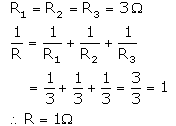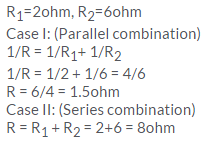Courses

# Solutions of Electricity (Page No- 37) - Physics By Lakhmir Singh, Class 10 Class 10 Notes | EduRev

## Class 10 : Solutions of Electricity (Page No- 37) - Physics By Lakhmir Singh, Class 10 Class 10 Notes | EduRev

The document Solutions of Electricity (Page No- 37) - Physics By Lakhmir Singh, Class 10 Class 10 Notes | EduRev is a part of the Class 10 Course Class 10 Physics Solutions By Lakhmir Singh & Manjit Kaur.
All you need of Class 10 at this link: Class 10

Lakhmir Singh Physics Class 10 Solutions Page No:37

Question 1:
Give the law of combination of resistances in series.
Solution :
According to the law of combination of resistances in series, the combined resistance of any number of resistances connected in series is equal to the sum of the individual resistances.

Question 2:
If five resistances, each of value 0.2 ohm, are connected in series, what will be the resultant resistance ?
Solution :
As per the law of combination of resistances in series,
R=R1+ R2+ R3+ R4+ R5
R=0.2+0.2+0.2+0.2+0.2=1ohm.

Question 3:
State the law of combination of resistances in parallel.
Solution :
According to the law of combination of resistance in parallel, the reciprocal of the combined resistance of a number of resistances connected in parallel is equal to the sum of the reciprocals of all the individual resistances.

Question 4:
If 3 resistances of 3 ohm each are connected in parallel, what will be their total resistance ?
Solution :Question 5:
How should the two resistances of 2 ohms each be connencted so as to produce an equivalent resistance of 1 ohm ?
Solution :
Since the resultant resistance is less than the individual resistances, so the resistances should be connected in parallel.

Question 6:
Two resistances X and Y are connected turn by turn : (i) in parallel, and (ii) in series. In which case the resultant resistance will be less than either of the individual resistances ?
Solution :
In case of parallel combination, the resultant resistance will be less than either of the individual resistances.

Question 7:
What possible values of resultant resistance one can get by combining two resistances, one of value 2 ohm and the other 6 ohm ?
Solution :Offer running on EduRev: Apply code STAYHOME200 to get INR 200 off on our premium plan EduRev Infinity!

94 docs

,

,

,

,

,

,

,

,

,

,

,

,

,

,

,

,

,

,

,

,

,

,

,

,

;